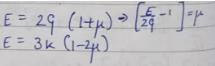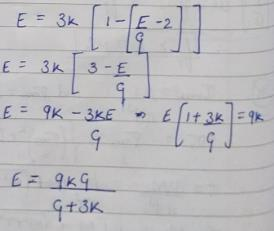Courses

# Test: Properties Of Metals, Simple Stress Strain And Elastic Constants - 2

## 10 Questions MCQ Test Topicwise Question Bank for GATE Civil Engineering | Test: Properties Of Metals, Simple Stress Strain And Elastic Constants - 2

Description
This mock test of Test: Properties Of Metals, Simple Stress Strain And Elastic Constants - 2 for Civil Engineering (CE) helps you for every Civil Engineering (CE) entrance exam. This contains 10 Multiple Choice Questions for Civil Engineering (CE) Test: Properties Of Metals, Simple Stress Strain And Elastic Constants - 2 (mcq) to study with solutions a complete question bank. The solved questions answers in this Test: Properties Of Metals, Simple Stress Strain And Elastic Constants - 2 quiz give you a good mix of easy questions and tough questions. Civil Engineering (CE) students definitely take this Test: Properties Of Metals, Simple Stress Strain And Elastic Constants - 2 exercise for a better result in the exam. You can find other Test: Properties Of Metals, Simple Stress Strain And Elastic Constants - 2 extra questions, long questions & short questions for Civil Engineering (CE) on EduRev as well by searching above.
QUESTION: 1

Solution:
QUESTION: 2

### Notched bar tests are frequently used for testing the

Solution:

Notched bar test- Impact Strength of a Material
Indentation Test- Hardness of a Material
The machinability rating is determined by measuring the weighted averages of the normal cutting speed, surface finish, and tool life for each material.
Salt Spray/Fog Test- Corrosion resistance of the material.

QUESTION: 3

### In the creep test, the following type of stress is applied to the specimen

Solution:
QUESTION: 4

A free bar of length / is uniformly heated from 0°C to a temperature t°C, α is the coefficient of linear expansion and E is the modulus of elasticity. The stress in the bar is

Solution:

Since the bar is free to expand, no stresses will be developed in the bar.

QUESTION: 5

A test specimen is stressed slightly beyond the yield point and then unloaded. Its yield strength

Solution: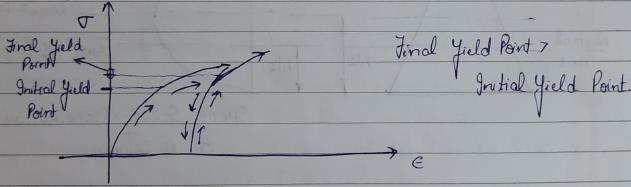QUESTION: 6

Cup-and-cone type fracture occurs in the case of

Solution:
QUESTION: 7

Materials having elongation less than 5% are considered brittle. In such cases, factor of safety is based on

Solution:
QUESTION: 8

A rod of length 'l’ and cross-sectional area ‘A’ rotates about an axis passing through one end of the rod. The extension produced in the rod due to centrifugal forces is (w is the weight of the rod per unit length and ω is the angular velocily of rotation of the rod)

Solution: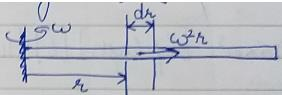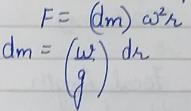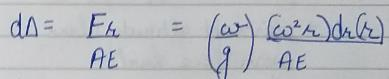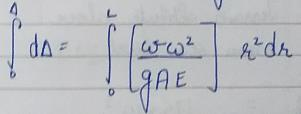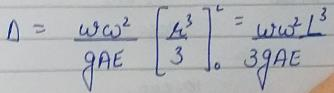QUESTION: 9

Young’s modulus of elasticity and Poisson’s ratio of a material are 1.25 x 105 MPa and 0.34 respectively. The modulus of rigidity of the material is

Solution: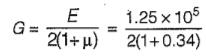= 0.4664 x 105 MPa

QUESTION: 10

In a homogeneous, isotropic elastic material, the modulus of elasticity E in terms of G and K is equal to

Solution: